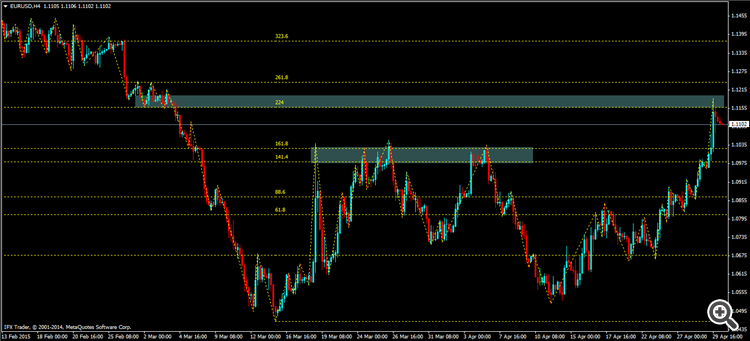# Support and Resistance based on Fibonacci Channel4
Fiboholic

Hope u all in the best condition ..

By the way .. i am a novice trader, and want to share simple things about support and resistance.

i was thinking that in order to find support and resistance we can use fibonacci channel.

Fibonacci Channels are built using several parallel trend lines. To build this instrument, the channel having the width taken as a unit width is used. Then, parallel lines are drawn at the values equal to the Fibonacci Numbers, beginning with 0.618-fold size of the channel, then 1.000-fold, 1.618-fold, 2.618-fold, 4.236-fold, etc. As soon as the fifth wave finishes, correction in the direction opposite to the trend can be expected.

It is necessary to remember for a correct Fibonacci Channel building: base line limits the upper part of the channel when trend is ascending, and the lower part of it when trend is descending. ( source : Mql4.com)

While in investopedia, it is said that  Fibonacci channel is a variation of the fibonacci retracement pattern in which the trendlines run diagonally rather that horizontally. These channels are used to estimate areas of support and resistance in the same way as the horizontal Fibonacci retracement levels.

Ok so.. instead of use  the channel diagonally, i use it horizontally. The reason is just because it looks easier to me :)

I use some of levels, which are 61.8, 88.6, 141.4, 161.8, 224, 261.8, 323.6. Below is the example on eurusd h4Here is another example, this time is on audusd h4

Ok thats all, its just an introduction, maybe some of traders interested with it, therefore we can discuss it later.

Thank you5

Damar Wahyujati:

Ayang...

Hope u all in the best condition ..

By the way .. i am a novice trader, and want to share simple things about support and resistance.

i was thinking that in order to find support and resistance we can use fibonacci channel.

Fibonacci Channels are built using several parallel trend lines. To build this instrument, the channel having the width taken as a unit width is used. Then, parallel lines are drawn at the values equal to the Fibonacci Numbers, beginning with 0.618-fold size of the channel, then 1.000-fold, 1.618-fold, 2.618-fold, 4.236-fold, etc. As soon as the fifth wave finishes, correction in the direction opposite to the trend can be expected.

It is necessary to remember for a correct Fibonacci Channel building: base line limits the upper part of the channel when trend is ascending, and the lower part of it when trend is descending. ( source : Mql4.com)

While in investopedia, it is said that  Fibonacci channel is a variation of the fibonacci retracement pattern in which the trendlines run diagonally rather that horizontally. These channels are used to estimate areas of support and resistance in the same way as the horizontal Fibonacci retracement levels.

Ok so.. instead of use  the channel diagonally, i use it horizontally. The reason is just because it looks easier to me :)

I use some of levels, which are 61.8, 88.6, 141.4, 161.8, 224, 261.8, 323.6. Below is the example on eurusd h4

Here is another example, this time is on audusd h4

Ok thats all, its just an introduction, maybe some of traders interested with it, therefore we can discuss it later.

Thank you